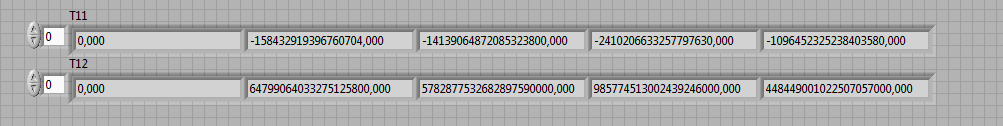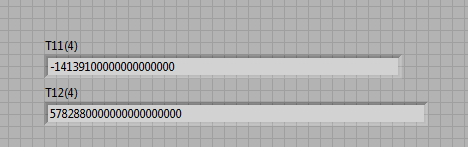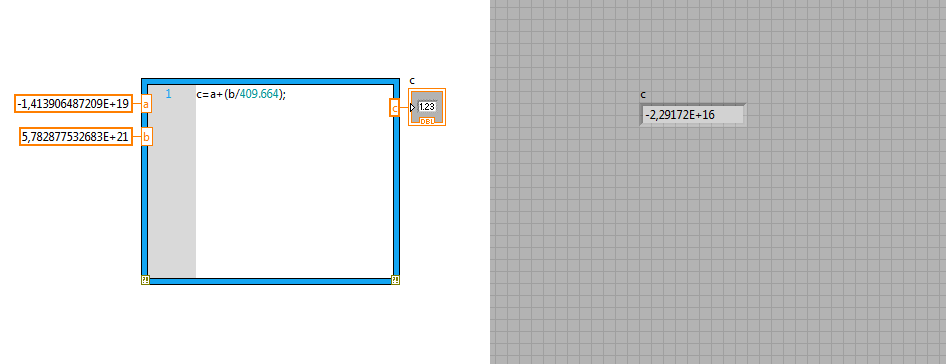# LabVIEW MathScript RT Module

cancel
Showing results for
Did you mean:

## Why does the MathScript calculates wrong?

Hi everyone,

I have two values obtained in the MathScrip and simply trying to get sum of them.

the first value is 1,640220000000000e+18

the seconds is  -1,640217348851742e+18

It should result as 2651148258304 but instead LabVIEW results that as zero. The data is represented in double precision and MATLAB result true when it is done inside it.

Why does it calculate wrong?

here is the code I'm using

regards,

ozgur

Message 1 of 6
(4,761 Views)

## Re: Why does the MathScript calculates wrong?

Your VI relies on more than a dozen input files that you have not attached. I cannot see your two constants that you want to add anywhere. A lot of your code also deals with complex data.

This is not helpful at all. please reduce the problem to a simple mathscript node with your two constants (mentioned in the first post) as inputs, producing the claimed wrong result.LabVIEW Champion. It all comes together inGCentralWhat does "Engineering Redefined" mean??
Message 2 of 6
(4,760 Views)

## Re: Why does the MathScript calculates wrong?

Altenbach,

I know it not helpful in that format, but there so many parameters that I couldn't simplify the process, sorry about that.

I've figured out why the problem is caused, simply let me explain.

The arrays (T11 and T12) that I obtain with MathScript operation is as below;I use the whole array, but lets assume that I use the 4th elements in those. The calculation is T11+(T12/409,664), It results approximately -2.2917e+016

But, when I write those 4th elements into .lvm file it rounds the data as below;Therefore, the calculation carries huge error. I think the Script makes the same approach when using these data. When I use the actual values as constants and put them into MathScript, it result exactly right;What to do to correct that ?

Regards,

Ozgur

Message 3 of 6
(4,757 Views)

## Re: Why does the MathScript calculates wrong?

Why don't you attach the simplified problem with the two arrays shown above. Make sure the inputs contain the correct values as default.

Do you think the problem is the calculation or the formatting into a lvm file? What format do you use?LabVIEW Champion. It all comes together inGCentralWhat does "Engineering Redefined" mean??
Message 4 of 6
(4,746 Views)

## Re: Why does the MathScript calculates wrong?

Altenbach,

When I create an array containing the data above and put into MathScript, it gives correct result; that's what I go crazy. I use text format .lvm when recording.

But when it is calculated and used in my actual code, I results wrong. ı mean the code that I first attached; which you couldn't use.

Also I use text format .lvm when recording. the data.

Ozgur

Message 5 of 6
(4,742 Views)

## Re: Why does the MathScript calculates wrong?

Then attach the lvm fine that is giving problems. How many significant digits does it contain (look at it in notepad!).LabVIEW Champion. It all comes together inGCentralWhat does "Engineering Redefined" mean??
Message 6 of 6
(4,738 Views)